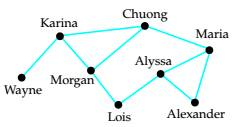# Degrees of Separation In the graph below, an edge connects two vertices if the corresponding people have communicated by text message. Here we define the degree of separation between two individuals to be the minimum number of steps required to link one person to another through text message communications. This is equivalent to the (minimum) number of edges in a path connecting the corresponding vertices. For example, Karina and Lois have a degree of separation of 2. a. What is the degree of separation between Morgan and Maria? b. What is the degree of separation between Wayne and Alexander? c. Who has the largest degree of separation from Alyssa?### Mathematical Excursions (MindTap C...

4th Edition
Richard N. Aufmann + 3 others
Publisher: Cengage Learning
ISBN: 9781305965584

#### Solutions

Chapter
Section### Mathematical Excursions (MindTap C...

4th Edition
Richard N. Aufmann + 3 others
Publisher: Cengage Learning
ISBN: 9781305965584
Chapter 5.1, Problem 31ES
Textbook Problem
38 views

## Degrees of Separation In the graph below, an edge connects two vertices if the corresponding people have communicated by text message. Here we define the degree of separation between two individuals to be the minimum number of steps required to link one person to another through text message communications. This is equivalent to the (minimum) number of edges in a path connecting the corresponding vertices. For example, Karina and Lois have a degree of separation of 2.a. What is the degree of separation between Morgan and Maria?b. What is the degree of separation between Wayne and Alexander?c. Who has the largest degree of separation from Alyssa?

To determine

(a)

To determine the degree of separation between Morgan and Maria.

### Explanation of Solution

Given information:

Given, in the graph below, an edge connects two vertices if the corresponding people have communicated by text message. The degree of separation between two individuals is the minimum number of steps required to link one person to another through text message communications which is same as the minimum number of edges in a path connecting the corresponding vertices.

To determine

(b)

To determine the degree of separation between Wayne and Alexander.

To determine

(c)

To determine the largest degree of separation from Alyssa.

### Still sussing out bartleby?

Check out a sample textbook solution.

See a sample solution

#### The Solution to Your Study Problems

Bartleby provides explanations to thousands of textbook problems written by our experts, many with advanced degrees!

Get Started

Find more solutions based on key concepts
Solve the differential equation. 8. y = y"

Calculus: Early Transcendentals

27r63s2t4

Applied Calculus for the Managerial, Life, and Social Sciences: A Brief Approach

Subtract 4x26x+2 from 4x2+6.

Elementary Technical Mathematics

Write each expression in terms of i. 21

Trigonometry (MindTap Course List)

The range of is: (−∞,∞) [0, ∞) (0, ∞) [1, ∞]

Study Guide for Stewart's Multivariable Calculus, 8th

The harmonic series is: 1 + 2 + 3 + 4 + …

Study Guide for Stewart's Single Variable Calculus: Early Transcendentals, 8th

If mn the number of integers from m to n inclusive is .

Discrete Mathematics With Applications

PRACTICE PROBLEMS Solve by factoring x28x=9.

Functions and Change: A Modeling Approach to College Algebra (MindTap Course List)